# WACC Adjustment to Correct Valuation of Tax Shields

This webpage explains how to adjust the WACC and the cost of equity to correctly reflect interest tax shields. The correct approach to valuation in the case with interest tax shields is to use net debt in the capital structure and apply non-tax adjusted cost of debt from the perspective of the investor. However I am not so naive as to believe anybody will actually use net debt in the capital structure as they should. Instead, I show on this webpage how you can adjust the WACC and the cost of equity to derive a case where the results are consistent with the correct net of tax case.

Reconciling the WACC to produce correct results in terms of valuation further confirms problems with valuation using the gross debt with reducing the debt for market value faced by the firm. Whenever the gross value of debt is used, the enterprise value is not correct and not consistent with the Ku and Bu. The only way to make the total capital (equity and debt) consistent with the true enterprise value that is defined as the Ku (without tax adjustments) is to adjust and change the Ku.  This adjustment to the overall cost of capital (WACC or Ku) cannot be explained by some simple tax adjustment to the cost of capital. Instead, the adjusted cost of capital must come from the backing out the cost of capital from the correct net of tax method. The file that includes analysis of reconciling the WACC with the tax shield can be downloaded by pressing the button below.

## Reconciliation of Net Debt and Ku or WACC

The reconciliation of net of tax capital structure and different WACC methods that apply the gross debt are shown in the screenshot below. The screenshot shows four different ways to compute the capital structure. The first capital structure shown in the screenshot uses the assumed debt that comes from the capital ratio and applies the net of tax method to establish the enterprise value and the equity value.  This was reconciled to net cash flow in the prior webpage. The second capital structure on the screenshot adds the tax shield to the net debt debt to establish the gross amount of debt that is typically included in the WACC or Ku analysis. In this case the pre-tax Ku is assumed not to change from the net capital structure case.  However, a tax effect of the interest rate is included and after-tax Ku applied to the free cash flows is reduced resulting in a value of 5.78% instead of 6.88%. The cost of equity is derived from the after-tax Ku and the after cost of debt. This results in a cost of equity of 8% in the example compared to a cost of equity of  7.57% in the net of tax case.  The cost of equity is also not consistent with the cash flow to equity as demonstrated below.

The third capital structure applies the cost of equity from the net debt method that in turn comes from the Bu, Be and Bd derived from the sample of companies. In this case the Ke comes is the same number as the Ke derived from the Bu and Ku analysis. With the Ke input, the Ku is derived. In this case, the Ku that is calculated does not produce that correct valuation of the enterprise value or the correct valuation of the equity cash flow.  I hope this demonstrates the importance of creating a cash flow model to verify and prove different valuation techniques.

The fourth capital structure reconciles the capital structure using gross debt and net of tax interest rates with the correct method where debt is measured net of tax and the debt cost of capital is at the pre-tax rate faced by investors. In this case the Ku is derived to produce the correct enterprise value and the cost of equity is backed out from the capital structure with net of tax interest rates.  Reconciling the Ku with the correct enterprise value is simple if the growth rate in cash flow after operating tax is constant. In this case the present value of equity cash flow discounted at the computed cost of equity produces the correct equity value.  Details of this case are presented as for the other methods.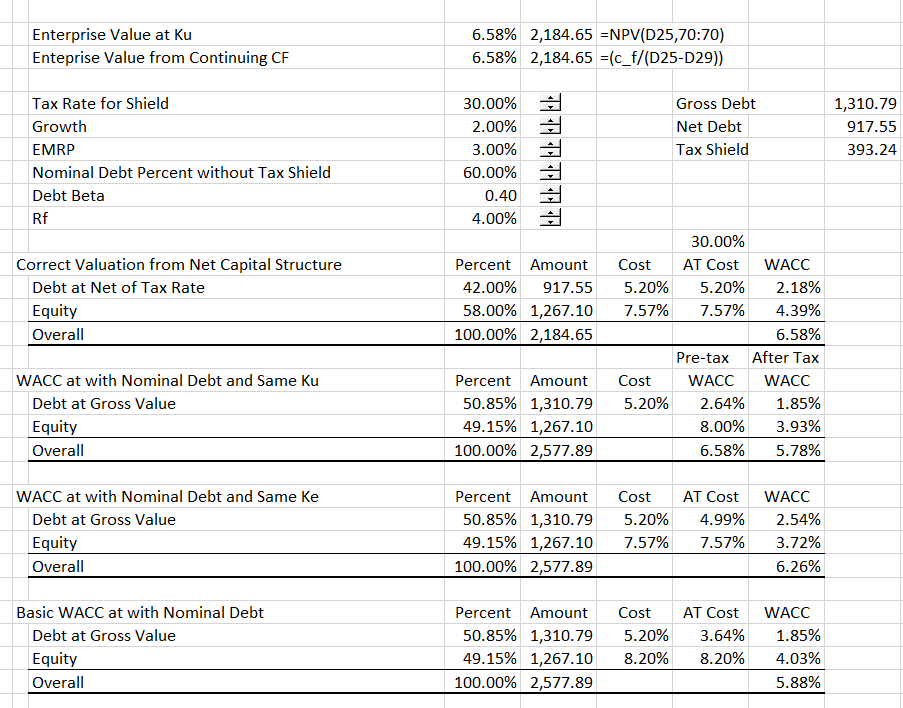## WACC with given Ku and Derived Cost of Equity Does Not Produce Correct Valuation

The screenshot below illustrates how valuation from the gross capital structure with the same Ku as the net of tax method does not produce correct valuation.  In reviewing this case please understand that Ku can be given by different formulas with tax and with different samples.  But just assume there is some correct Ku to apply to free cash flow in establishing the enterprise value. That’s all that is important — that there is some enterprise value that comes from free cash flow and the risk applied to this cash flow. Whether this free cash flow is financed with subsidized debt, equity or different levels of debt does not matter to the ultimate enterprise.  Come on.  This is what Merton Miller got correct.  The value of the enterprise or the house or your I-phone or your Lambrigini does not have anything to do with whether you buy with a credit card or a long-term loan and even whether you get subsidized financing. Why do finance academics like Damarodan and Myers have so much problem with this simple idea.

If the enterprise value is independent of the financing including the subsidized financing, then if you use the gross of tax capital structure, the sum of gross debt and the equity value does not equal the true enterprise value.  The addition of gross debt and the value of equity does not add up to enterprise value.  The gross debt includes the tax shield which means the enterprise value includes the tax shield. If the after-tax free cash flow is discounted at the Ku, the present value of cash flow cannot be correct because it should not be distorted by the tax shield.  The tax shield is something that is transferred between the entity that provides the subsidy and the equity holder. I have to re-emphasize this point.

The target enterprise value when using the gross of tax capital structure is higher than the enterprise value when using the net of tax debt value.  This is because the gross tax amount that does not reflect the interest tax shield is used as a subtraction to arrive at the equity value.  In the screenshot below the difference between using net of tax and gross of tax is illustrated.  As the debt is increased, the enterprise value must also be increased so that that equity value will remain correct. In the screenshot the difference between both the debt and the enterprise value is the value of the tax shield which is 393.24.If the case where the Ku is taken from the sample then adjusted for tax, the Ku falls from 6.88% in the net of tax case without the after-tax interest rate to 5.78% with the after-tax interest cost. The decline in the all-equity cost of capital from 6.88% to 5.78% increases enterprise value. This decline in the cost of capital which accounts for the additional tax shield that is added to capital. But the increase in the enterprise value above the net of tax amount is not accurate.  The magnitude of the error from using traditional WACC with gross debt depends on the growth rate assumed.  With low cash flow growth rates, the error may be relatively small (you can experiment with the growth rate in the file for download above).  However, with high growth rates in cash flow and low cost of capital, the error can be large. In the case shown on the screenshot below that assumes a 4% growth in free cash flow, the error in measuring equity value and the the enterprise value is 22%. You can see this in the second column below. The enterprise value is 5604 instead of 4578 and the equity value is 2,755 instead of 2,250.  The errors would be produces if the gross of tax method were used with the free cash flow that grows at 4%.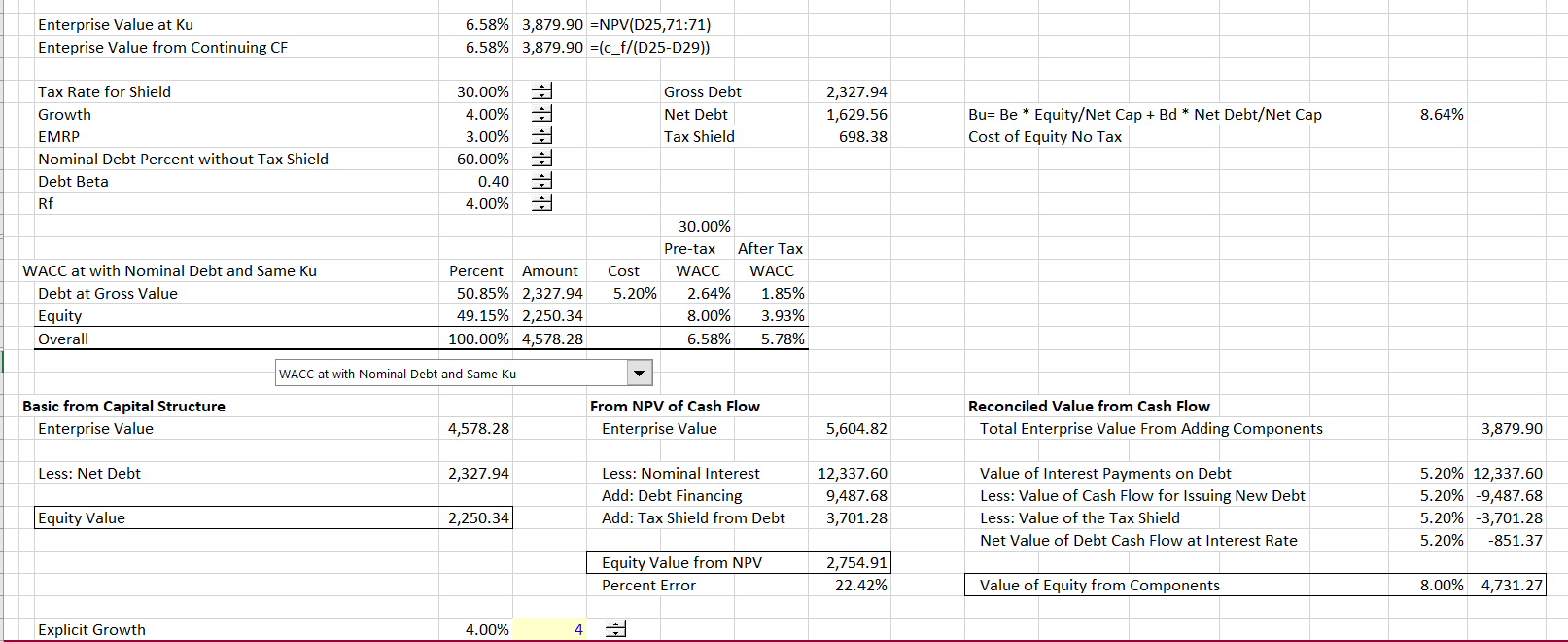Details of how the equity value, debt value and enterprise value are computed from cash flow and the cost of capital in the gross debt capital structure is shown in the next screenshot.  The screenshot demonstrates that with a relatively high growth rate, the discounted cash flow model using continuing cash flow produces enterprise value of 5,604 from the net present value of cash flow. The difference of 22% from the present cash flow at the Ku can be explained by dividing 100 by the Ku of 5.78%. If the discounted cash flow model is used, this error will be made and the value will be incorrect.  In addition the equity value computed from either the equity cash flow or the enterprise value less the value of the debt will have the same error.  This is demonstrated in screenshot.## WACC with given Ke and Derived Overall WACC Does Not Produce Correct Valuation

Instead of computing the same all-equity cost of capital (adjusted for tax on the cost of debt) with traditional WACC that uses the gross non tax adjusted debt, a capital structure that includes gross debt and the same cost of equity as the net debt capital structure is evaluated.  The capital structure with the net of tax debt capital structure assumes a cost of equity of 7.57%. In the traditional WACC capital structure, this same cost of equity is used. But the debt is measured at the gross of tax amount (i.e. without multiplying by 1-t) and net of tax interest rate. With the alternative debt to capital ratio and the net of tax interest rate, the overall cost of capital becomes 5.57%.  The 5.57% compares to the 6.88% all-equity cost of capital in the net of tax case. When this 5.57% cost of capital is used to measure the value of cash flow, the value is not the correct enterprise value.  Similarly, the equity value from the measured equity cash flow at the 7.57% cost of capital produces errors.  This is shown in the screenshot below. The implication of this error is that when the gross of tax debt capital structure is used with the cost of equity from an analysis of comparative companies, the result is not correct.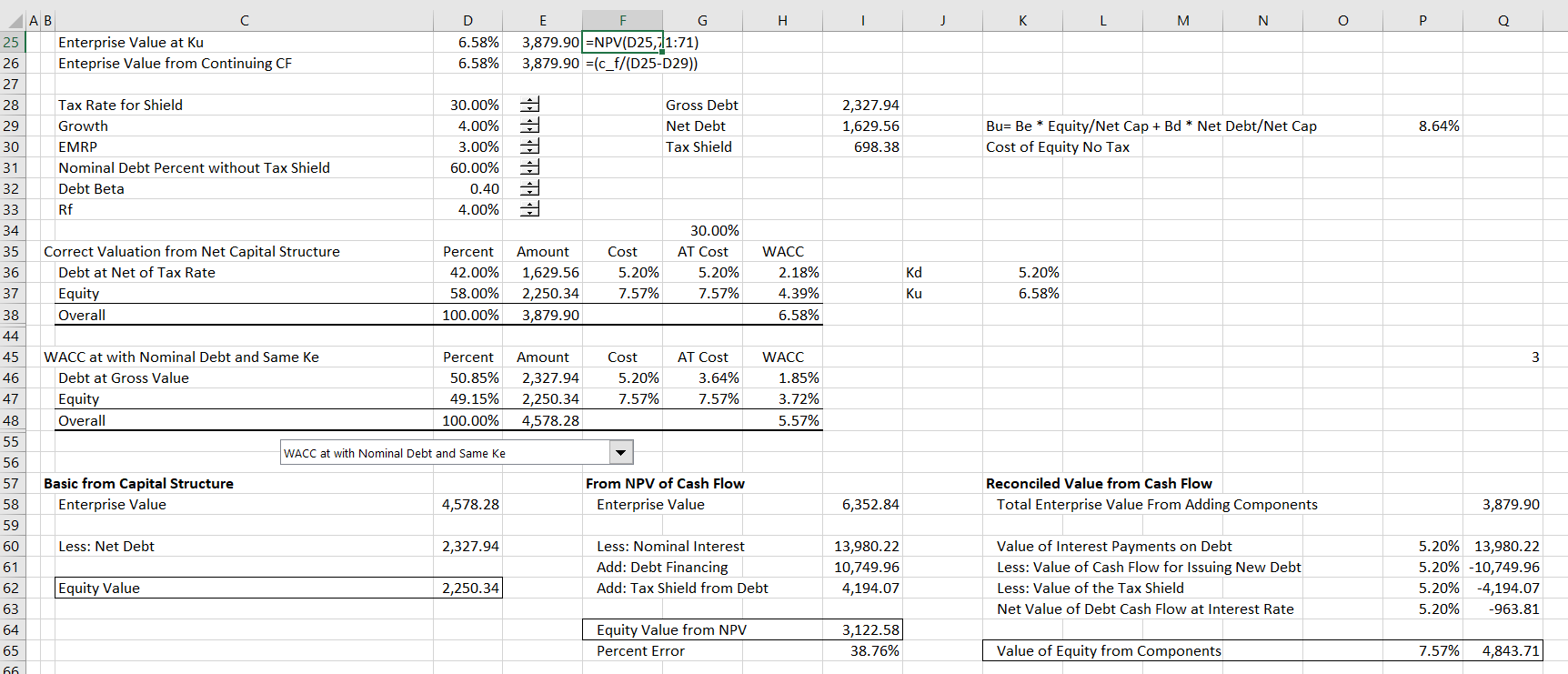The error for a growth rate of 2% is shown in the screenshot below. In this case the error is lower, which is explained by the fact that Value = CF/(Ku-g).  With lower growth, the denominator is higher and the value is higher.  In this case the error from using the debt that is gross of tax and the traditional WACC is shown below.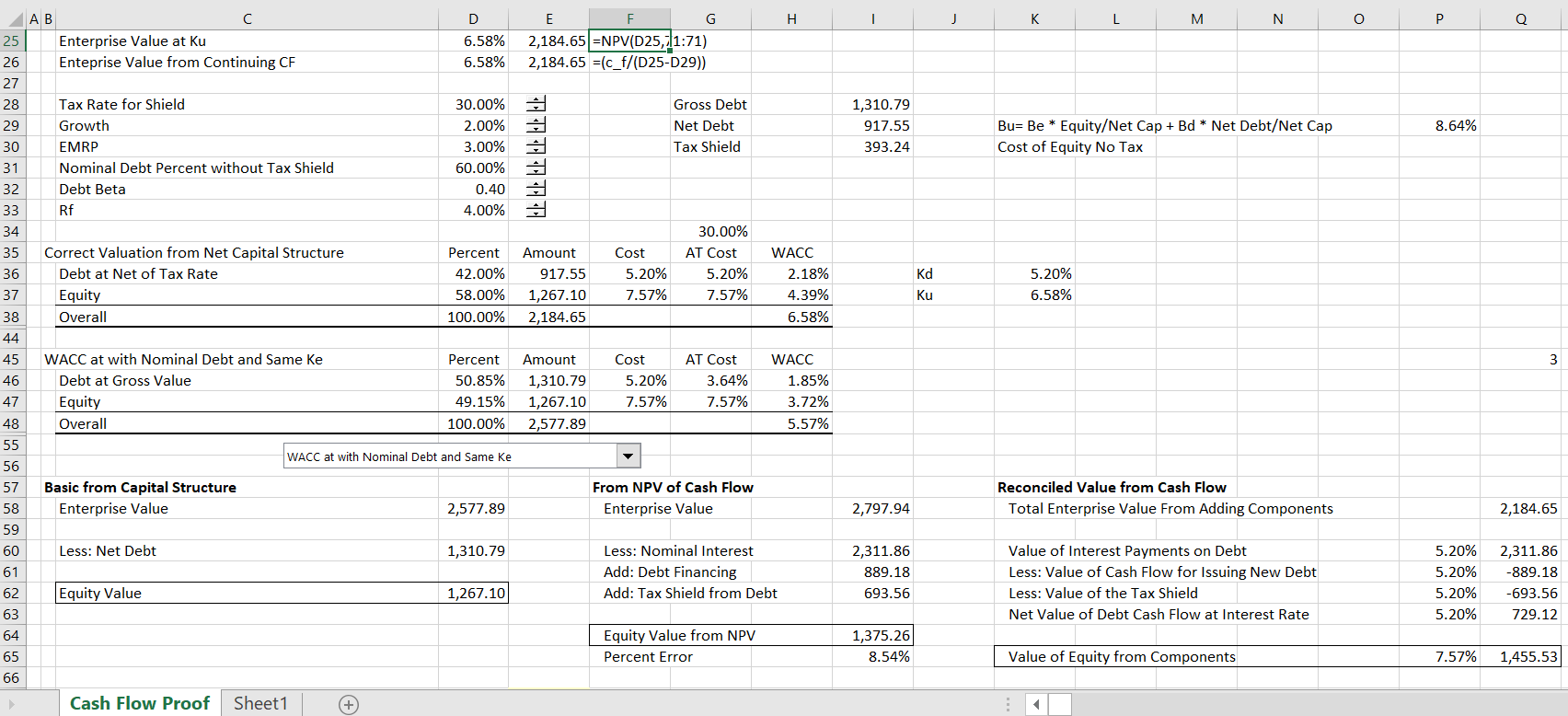## Reconciling WACC and Ke to Yield Correct Valuation Consistent with Net Debt Method

The traditional WACC method or the Ku can be reconciled with the net of tax debt method by deriving cost of capital that produces a correct enterprise value.  The enterprise value target from a traditional gross of tax capital structure must include the tax effect of debt meaning that the enterprise value from the net debt capital structure is increased for the tax effect.  In order to derive this enterprise value plus tax shield, an implied WACC or Ku can be computed.  Once the Ku or WACC is computed, the gross of tax debt can be put in the capital structure and the cost of equity can be derived.  The cost of equity is computed from the overall WACC less the debt percent multiplied by the after-tax cost of debt. Cost of equity is then the difference divided by the cost of equity.

A summary of backing into WACC that reconciles to the enterprise value including the tax shield involves the following three steps. Note that the calculation requires first establishing the enterprise value from the net of tax debt method.

1. Compute Target EV plus tax shield from EV + Tax Shield in the net of tax method
2. Compute Implied Ku: Value = CF/(k-g); k-g = CF/Value; k = CF/Value + g
3. Compute Implied Ke from (Ku – Debt/Capital * After-tax Interest)/(Equity/Capital)

Results of this reconciliation method are shown in the screenshot below. Note that the Ku is now 6.18% which is not as low as the other traditional WACC cases. With this WACC and the net of tax interest rate, the implied cost of equity is 8.82%.  With this cost of overall capital and cost of equity capital the results match the net of tax debt method.  This is shown in the second column of the screenshot below.When this reconciled cost of capital is applied to cash flow, the valuation produces the correct result.  This is demonstrated in the screenshot below. The reconciliation process demonstrates that the net of tax method is the only method that produces correct valuation.  If you want to reconcile the valuation, you need to start with the net of tax debt.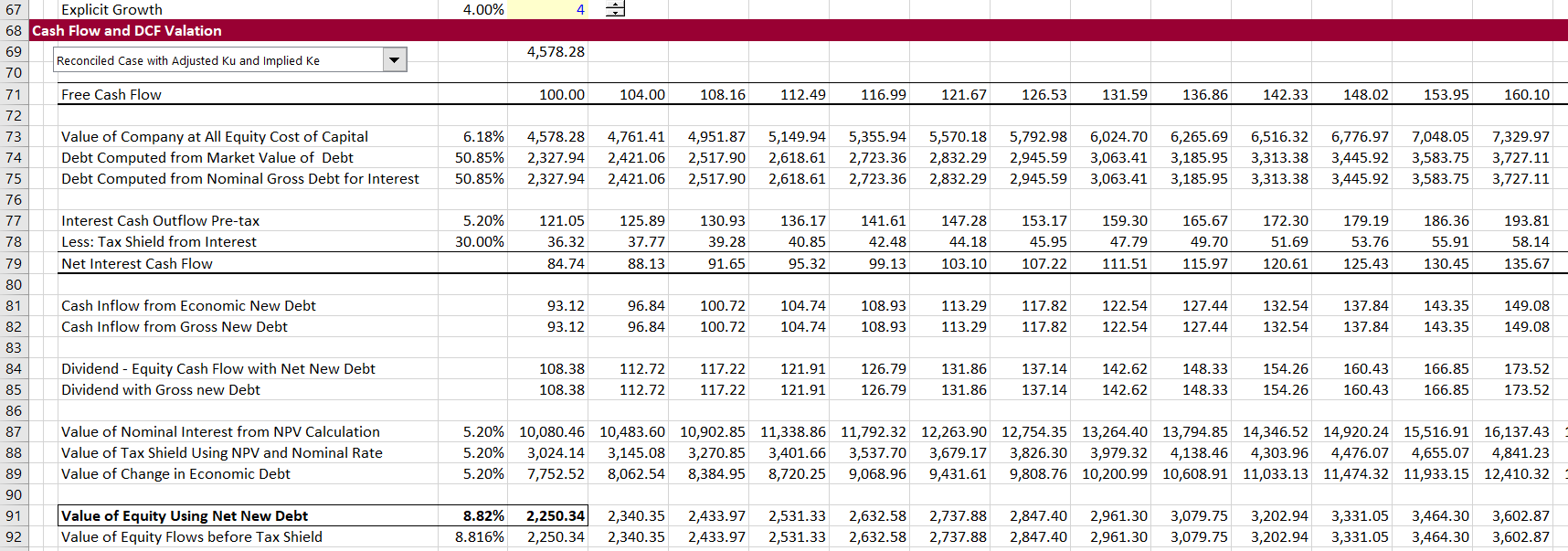## Video Explanations

Video explanations include theory and some background APV does not add anything new to valuation. Correct application of the WACC accounts for all items in the bridge between equity value and enterprise value in the WACC calculation. Investment bankers typically do this with using net debt rather than gross debt in computing WACC. Similar adjustments to the WACC should be made for associated investments not included in EBITDA, the fair value of derivatives, discounted operations and any other items.